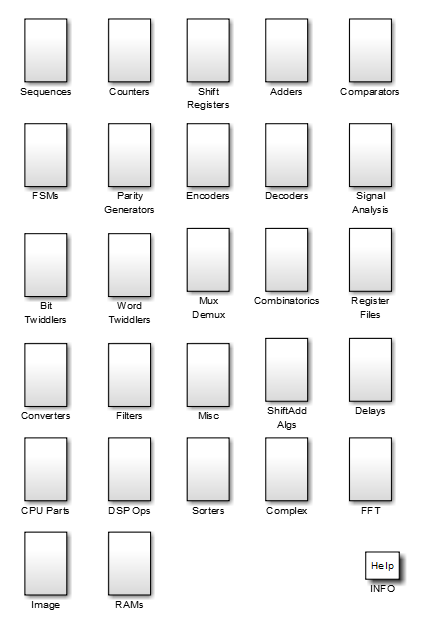## MATLAB Function Block Design Patterns for HDL

You can use MATLAB Function blocks to write algorithms for implementation in Simulink® and Stateflow®, then use this code for HDL code generation. You can use the HDL design patterns library `eml_hdl_design_patterns` to implement common hardware modeling algorithms using the MATLAB Function block. The library contains examples that demonstrate hardware-friendly applications of the MATLAB Function block for HDL code generation.To open the library, at the MATLAB® command prompt, enter:

`eml_hdl_design_patterns`

You can use the blocks in the library to model various hardware elements by:

• Copying a block from the library to your model and using it as a computational unit.

• Copying the code from the block and using it as a local function in an existing MATLAB Function block.

When you create custom blocks, you can control whether to inline or instantiate the HDL code generated from MATLAB Function blocks. To inline or instantiate the HDL code, in the Configuration Parameters dialog box, click HDL Code Generation > Global Settings. In the right pane, in the Coding style tab, select Inline MATLAB Function block code.

Note

Do not use the Inline MATLAB Function block code setting when you set the Architecture HDL block property of the MATLAB Function block to `MATLAB Datapath`. Enable FlattenHierarchy instead. For more information, see HDL Optimizations Across MATLAB Function Block Boundary Using MATLAB Datapath Architecture.

### HDL Design Pattern Blocks

This table summarizes some of the categories of HDL design patterns in the `eml_hdl_design_patterns` library. For more information on the different blocks, see Create Hardware Design Patterns Using the MATLAB Function Block For HDL Code Generation.

Library SubsystemPurpose
`Combinatorics`

Combinatorial fixed-point algorithms, such as expressions containing addition, subtraction, and multiplication operators with different fixed-point data types.

`Adders`

Algorithms that model different adder logic.

`Delays`Algorithms to model integer delay, tap delay, and tap delay vector blocks by using vectors of persistent variables. Use these design patterns to implement sequential algorithms that carry state between executions of the MATLAB Function block in a model.
`Comparators`Algorithms for finding the minimum value of a vector.
`FSMs`

MATLAB Function block control constructs such as `switch/case` and `if-else`, coupled with fixed point arithmetic operations to model control logic quickly.

The `FSMs/mealy_fsm_blk` and `FSMs/moore_fsm_blk` blocks provide example implementations of Mealy and Moore finite state machines in the MATLAB Function block.

`Counters`

Counter logic that shows how to model state and quantize data elements within loops.

`Shift Registers`

Algorithms that model shift register hardware elements.

The `shift_reg_1by32` block shows how to model shift registers by using the `bitsliceget` and `bitconcat` functions. This function implements a serial input and output shifters with a 32–bit fixed-point operand input.

The `shift_reg_1by64` block shows a 64 bit shifter. In this case, the shifter uses two fixed-point words to represent the operand, overcoming the 32-bit word length limitation for fixed-point integers.

`Word Twiddlers`Algorithms that perform word conversions, such as conversions from an integer to bits and bits to an integer.

### Using Blocks in this Library for HDL Code Generation

To build models using blocks in this library and generate HDL code:

• Create or open a model.

• Copy a block from `eml_hdl_design_patterns` library to your model.

• Place the block in the device under test (DUT) subsystem.

• Run the `hdlsetup` command to set up your model for HDL code generation.

• Run the `makehdl` function to generate HDL code for the DUT subsystem.

For more information on generating HDL code from a MATLAB Function block, see Generate HDL Code from a MATLAB Function Block.

### Fixed-Point Algorithm Support

The MATLAB Function block supports floating-point arithmetic and fixed-point arithmetic by using the Fixed-Point Designer™ `fi` function. This function supports rounding and saturation modes that you can use to code algorithms that manipulate arbitrary word and fraction lengths. HDL Coder™ supports all `fi` rounding and overflow modes. HDL code generated from the MATLAB Function block is bit-true to MATLAB semantics. Generated code uses bit manipulation and bit access operators, such as slice, extend, reduce, and concatenate that are native to VHDL and Verilog. The `eml_hdl_design_patterns` library includes example blocks that use the `fi` object, such as the blocks in the `Adders` subsystem.